Forum: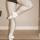Need help with a fairly straightforward Math 11 concept.
By Coccinella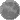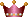On Fri Oct 05, 2012 12:05 PM
Edited by Coccinella (54968) on 2012-10-05 12:11:10

Write the equation of the line in slope-intercept form using the given information.

Question #13) (2,7); m = 2/3

So, I know I need to use y=mx+b form in this equation and that b = the place where the line crosses the y-axis and that the mx part is the slope. With this particular problem, I can't for the life of me seem to figure out the b part. If I go over 2 on the x axis and up 7 on the y axis I'm nowhere near the y axis when I plot the next point using the slope. Any help for me here?

Thanks!

### 5 Replies to Need help with a fairly straightforward Math 11 concept.re: Need help with a fairly straightforward Math 11 concept. (karma: 1)
By ChristineOn Fri Oct 05, 2012 06:36 PM
I used to make myself a little "chart".

When "x" is ____
then "y" is____

Plug the variables into this and you will have several points along your line. You really only need two points determine a line but a few "extras" will help you to check your findings.

In the example you gave, I assume the (2,7) mean when x is 2, y is 7. With a slope (m) of 2/3, that means the ratio of the change in y, over the change in x, is "two over, three up", in your terms, not 7.

Hope this helps.

Keep On Dancing*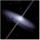re: Need help with a fairly straightforward Math 11 concept. (karma: 1)
By chica3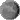On Fri Oct 05, 2012 02:18 PM
Edited by chica3 (81066) on 2012-10-05 14:20:14
If I am correct in thinking that (2,7) is a point on the line, you can just write

y = mx + b
Given that m = 2/3, we know immediately that the equation is of the form:
y = (2/3)*x + b
Because we want the line to pass through (2,7) we can substitute x = 2 and y = 7 to solve for b:
7 = 2*(2/3) + b

Alternatively, to use your reasoning in case it's helpful: let's go to the point (2,7). We know that because m is positive the line slopes upwards (like /). Therefore to get closer to the y intercept (which is the point where x = 0), we need to move 2 units to the left. For every 3 units along the x axis, we move 2 units along the y axis:

3 units in -x direction (left) = 2 units in -y direction (down)
So:
2 units in -x direction = (2/3)*2 units in the -y direction (the y intercept).re: Need help with a fairly straightforward Math 11 concept.
By CoccinellaOn Fri Oct 05, 2012 02:56 PM
Edited by Coccinella (54968) on 2012-10-05 15:09:03
When I read both your answers it felt very clear to me but now I'm sitting with my math work and I'm getting increasingly confused.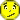I've plotted out a few different points so I can draw myself a complete line: I came up with X: 2,0,-2 and Y: 7,5,3. I plotted those points. But, I must have messed that up because when I count "up and over" to check to see if I have the rise/run correct, I don't. When I switched the points to be where they would be if I counted from the original 2,7 point than that seems right but now my Y-intercept doesn't cross the line cleanly...it's a fraction where the line crosses Y.

Sigh. No wonder it's taking me 12 months to do a high school level course as a 23 year old.

EDIT: Is this the correct response: y=2/3x+5 2/3 ?
re: Need help with a fairly straightforward Math 11 concept.
By wtalon
On Tue Oct 09, 2012 12:33 AM
No matter what form your teacher would like the equation to be in, when you see a point and a slope, there is almost one equation that should almost immediately come to mind- point-slope form. I can't imagine a time when you wouldn't use this equation, given a point and a slope.

Point-slope equation is for the given slope m and the point (a,c):

y-c=m(x-a)

At this point, you can just solve into 'y=mx+b' form by distributing the slope and simplifying like terms.

In your particular problem, the slope is 2/3 and the point is (2,7)/

Remember, it's okay to start off using a different form of the equation as long as you end up with the correct form of the equation.